# statistical mechanics

Also found in: Dictionary, Thesaurus, Wikipedia.

## statistical mechanics,

quantitative study of systems consisting of a large number of interacting elements, such as the atoms or molecules of a solid, liquid, or gas, or the individual quanta of light (see photonphoton
, the particle composing light and other forms of electromagnetic radiation, sometimes called light quantum. The photon has no charge and no mass. About the beginning of the 20th cent.
) making up electromagnetic radiation. Although the nature of each individual element of a system and the interactions between any pair of elements may both be well understood, the large number of elements and possible interactions can present an almost overwhelming challenge to the investigator who seeks to understand the behavior of the system. Statistical mechanics provides a mathematical framework upon which such an understanding may be built. Since many systems in nature contain large number of elements, the applicability of statistical mechanics is broad. In contrast to thermodynamicsthermodynamics,
branch of science concerned with the nature of heat and its conversion to mechanical, electric, and chemical energy. Historically, it grew out of efforts to construct more efficient heat engines—devices for extracting useful work from expanding hot gases.
, which approaches such systems from a macroscopic, or large-scale, point of view, statistical mechanics usually approaches systems from a microscopic, or atomic-scale, point of view. The foundations of statistical mechanics can be traced to the 19th-century work of Ludwig Boltzmann, and the theory was further developed in the early 20th cent. by J. W. Gibbs. In its modern form, statistical mechanics recognizes three broad types of systems: those that obey Maxwell-Boltzmann statistics, those that obey Bose-Einstein statisticsBose-Einstein statistics,
class of statistics that applies to elementary particles called bosons, which include the photon, pion, and the W and Z particles. Bosons have integral values of the quantum mechanical property called spin and are "gregarious" in the sense that an
, and those that obey Fermi-Dirac statisticsFermi-Dirac statistics,
class of statistics that applies to particles called fermions. Fermions have half-integral values of the quantum mechanical property called spin and are "antisocial" in the sense that two fermions cannot exist in the same state.
. Maxwell-Boltzmann statistics apply to systems of classical particles, such as the atmosphere, in which considerations from the quantum theoryquantum theory,
modern physical theory concerned with the emission and absorption of energy by matter and with the motion of material particles; the quantum theory and the theory of relativity together form the theoretical basis of modern physics.
are small enough that they may be ignored. The other two types of statistics concern quantum systems: systems in which quantum-mechanical properties cannot be ignored. Bose-Einstein statistics apply to systems of bosons (particles that have integral values of the quantum mechanical property called spin); an unlimited number of bosons can be placed in the same state. Photons, for instance, are bosons, and so the study of electromagnetic radiation, such as the radiation of a blackbodyblackbody,
in physics, an ideal black substance that absorbs all and reflects none of the radiant energy falling on it. Lampblack, or powdered carbon, which reflects less than 2% of the radiation falling on it, crudely approximates an ideal blackbody; a material consisting of a
involves the use of Bose-Einstein statistics. Fermi-Dirac statistics apply to systems of fermions (particles that have half-integral values of spin); no two fermions can exist in the same state. Electrons are fermions, and so Fermi-Dirac statistics must be employed for a full understanding of the conduction of electrons in metals. Statistical mechanics has also yielded deep insights in the understanding of magnetismmagnetism,
force of attraction or repulsion between various substances, especially those made of iron and certain other metals; ultimately it is due to the motion of electric charges.
, phase transitions, and superconductivitysuperconductivity,
abnormally high electrical conductivity of certain substances. The phenomenon was discovered in 1911 by Heike Kamerlingh Onnes, who found that the resistance of mercury dropped suddenly to zero at a temperature of about 4.
.

## Statistical mechanics

That branch of physics which endeavors to explain the macroscopic properties of a system on the basis of the properties of the microscopic constituents of the system. Usually the number of constituents is very large. All the characteristics of the constituents and their interactions are presumed known; it is the task of statistical mechanics (often called statistical physics) to deduce from this information the behavior of the system as a whole.

#### Scope

Elements of statistical mechanical methods are present in many widely separated areas in physics. For instance, the classical Boltzmann problem is an attempt to explain the thermodynamic behavior of gases on the basis of classical mechanics applied to the system of molecules.

Statistical mechanics gives more than an explanation of already known phenomena. By using statistical methods, it often becomes possible to obtain expressions for empirically observed parameters, such as viscosity coefficients, heat conduction coefficients, and virial coefficients, in terms of the forces between molecules. Statistical considerations also play a significant role in the description of the electric and magnetic properties of materials. See Boltzmann statistics, Intermolecular forces, Kinetic theory of matter

If the problem of molecular structure is attacked by statistical methods, the contributions of internal rotation and vibration to thermodynamic properties, such as heat capacity and entropy, can be calculated for models of various proposed structures. Comparison with the known properties often permits the selection of the correct molecular structure.

Perhaps the most dramatic examples of phenomena requiring statistical treatment are the cooperative phenomena or phase transitions. In these processes, such as the condensation of a gas, the transition from a paramagnetic to a ferromagnetic state, or the change from one crystallographic form to another, a sudden and marked change of the whole system takes place. See Phase transitions

Statistical considerations of quite a different kind occur in the discussion of problems such as the diffusion of neutrons through matter. In this case, the probability of the various events which affect the neutron are known, such as the capture probability and scattering cross section. The problem here is to describe the physical situation after a large number of these individual events. The procedures used in the solution of these problems are very similar to, and in some instances taken over from, kinetic considerations. Similar problems occur in the theory of cosmic-ray showers.

It happens in both low-energy and high-energy nuclear physics that a considerable amount of energy is suddenly liberated. An incident particle may be captured by a nucleus, or a high-energy proton may collide with another proton. In either case, there is a large number of ways (a large number of degrees of freedom) in which this energy may be utilized. To survey the resulting processes, one can again invoke statistical considerations. See Scattering experiments (nuclei)

Of considerable importance in statistical physics are the random processes, also called stochastic processes or sometimes fluctuation phenomena. The brownian motion, the motion of a particle moving in an irregular manner under the influence of molecular bombardment, affords a typical example. The stochastic processes are in a sense intermediate between purely statistical processes, where the existence of fluctuations may safely be neglected, and the purely atomistic phenomena, where each particle requires its individual description. See Brownian movement

All statistical considerations involve, directly or indirectly, ideas from the theory of probability of widely different levels of sophistication. The use of probability notions is, in fact, the distinguishing feature of all statistical considerations.

#### Methods

For a system of N particles, each of the mass m, contained in a volume V, the positions of the particles may be labeled x1, y1, z1, …, xN, yN, zN, their cartesian velocities vx1, …, vzN, and their momenta Px1, …, PzN. This simplest statistical description concentrates on a discussion of the distribution function f(x,y,z;vx,vy,vz;t). The quantity f(x,y,z;vx,vy,vz;t) &cdot; (dxdydzdvxdvydvz) gives the (probable) number of particles of the system in those positional and velocity ranges where x lies between x and x + dx; vx between vx and vx + dvx, and so on. These ranges are finite.

Observations made on a system always require a finite time; during this time the microscopic details of the system will generally change considerably as the phase point moves. The result of a measurement of a quantity Q will therefore yield the time average, as in Eq. (1). The integral is along the trajectory

(1)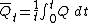in phase space; Q depends on the variables x1, …, PzN, and t. To evaluate the integral, the trajectory must be known, which requires the solution of the complete mechanical problem.

Ensembles. J. Willard Gibbs first suggested that instead of calculating a time average for a single dynamical system, a collection of systems, all similar to the original one, should instead be considered. Such an ensemble of systems is to be constructed in harmony with the available knowledge of the single system, and may be represented by an assembly of points in the phase space, each point representing a single system. If, for example, the energy of a system is precisely known, but nothing else, the appropriate representative example would be a uniform distribution of ensemble points over the energy surface, and no ensemble points elsewhere. An ensemble is characterized by a density function ρ(x1, …,zN; px1, …,pzN;t) ≡ p(x,p,t). The significance of this function is that the number of ensemble systems dNe contained in the volume element dx1dzN; dpxdpzN of the phase space (this volume element will be called dΓ) at time t is as given in Eq. (2).

(2)The ensemble average of any quantity Q is given

(3)by Eq. (3). The basic idea now is to replace the time average of an individual system by the ensemble average, at a fixed time, of the representative ensemble. Stated formally, the quantity defined by Eq. (1), in which no statistics is involved, is identified with defined by Eq. (3), in which probability assumptions are explicitly made.

Relation to thermodynamics. It is certainly reasonable to assume that the appropriate ensemble for a thermodynamic equilibrium state must be described by a density function which is independent of the time, since all the macroscopic averages which are to be computed as ensemble averages are time-independent.

The so-called microcanonical ensemble is defined by Eq. (4a), where c is a constant, for the energy E between E0 and E0 + ΔE; for other energies Eq. (4b)

(4{\it a})(4{\it b})holds. By using Eq. (3), any microcanonical average may be calculated. The calculations, which involve integrations over volumes bounded by two energy surfaces, are not trivial. Still, many of the results of classical Boltzmann statistics may be obtained in this way. For applications and for the interpretation of thermodynamics, the canonical ensembles is much more preferable. This ensemble describes a system which is not isolated but which is in thermal contact with a heat reservoir.

There is yet another ensemble which is extremely useful and which is particularly suitable for quantum-mechanical applications. Much work in statistical mechanics is based on the use of this so-called grand canonical ensemble. The grand ensemble describes a collection of systems; the number of particles in each system is no longer the same, but varies from system to system. The density function p(N,p,x) dΓN gives the probability that there will be in the ensemble a system having N particles, and that this system, in its 6N-dimensional phase space ΓN, will be in the region of phase space dΓN.

McGraw-Hill Concise Encyclopedia of Physics. © 2002 by The McGraw-Hill Companies, Inc.
The following article is from The Great Soviet Encyclopedia (1979). It might be outdated or ideologically biased.

## Statistical Mechanics

(or statistical physics), the branch of physics whose task is to express the properties of macroscopic substances—that is, systems consisting of a very large number of identical particles, such as molecules, atoms, or electrons—in terms of the properties of the particles and the interaction between the particles.

Macroscopic substances are also studied by other branches of physics, such as thermodynamics, continuum mechanics, and the electrodynamics of continuous media. When, however, specific problems are solved by the methods of these disciplines, the corresponding equations always contain unknown parameters or functions that characterize the given substance. For example, in order to solve hydrodynamic problems, it is necessary to know the equation of state of a liquid or gas—that is, the dependence of density on temperature and pressure, the specific heat of the fluid, the fluid’s viscosity, and other factors. Since all these functions and parameters can be determined experimentally, the methods being discussed are phenomenological in nature. By contrast, statistical mechanics, at least in principle (and sometimes in practice) permits these quantities to be calculated if the interaction forces between the molecules are known. Thus, statistical mechanics makes use of information on the microscopic structure of substances—that is, information on what particles the substances consist of and on how the particles interact. For this reason, statistical mechanics is referred to as a microscopic theory.

Suppose that the coordinates and velocities of all particles of a substance at some instant in time are given and that the law governing the interaction of the particles is known. In theory, the equations of mechanics can then be solved in order to find the coordinates and velocities at any subsequent time and thus to define completely the state of the system under study. (For simplicity, the language of classical mechanics is used here, but the situation is the same in quantum mechanics: if the initial wave function of a system and the law governing the interaction of the system’s particles are known, it is possible, by solving the Schrödinger equation, to find a wave function that defines the state of the system at all future times.) In actuality, however, a microscopic theory cannot be constructed in this way, since the number of particles in macroscopic substances is very great. For example, 1 cm3 of a gas at a temperature of 0°C and a pressure of 1 atmosphere contains approximately 2.7 × 1019 molecules. It is impossible to solve such a large number of equations, and, in any case, the initial coordinates and velocities of the molecules are unknown.

It is, however, precisely the large number of particles in macroscopic substances that leads to the appearance of new, statistical regularities in the behavior of such substances. Within broad limits the behavior is independent of the specific initial conditions—that is, of the exact values of the initial coordinates and velocities of the particles. The most important manifestation of this independence is the experimentally known fact that a system left to itself—that is, a system isolated from external factors—in time reaches an equilibrium state (thermodynamic, or statistical, equilibrium) whose properties are determined solely by such general characteristics of the initial state as the number of particles and their total energy (seeEQUILIBRIUM, THERMODYNAMIC). The following discussion deals primarily with the statistical mechanics of equilibrium states.

Before a theory describing the statistical regularities can be formulated, the requirements made of the theory should be delimited in a reasonable manner. Specifically, the objective of the theory should be to calculate not the exact values of various physical quantities for macroscopic substances but the average values of the quantities over time. Let us consider, for example, the molecules in some sufficiently large, or macroscopic, volume in a gas. Because of their motion, the number of molecules varies over the course of time. If the coordinates of the molecules at all times were known, the number could be found exactly. It is not, however, necessary to find the exact number. The change in the number of molecules in the volume has the character of random fluctuations about some average value. When the number of particles in the volume is large, the fluctuations are small in comparison with the average number of particles. Thus, it is sufficient to know this average value in order to characterize a macroscopic state.

The nature of the statistical regularities can be clarified by considering another simple example. Suppose two kinds of grain are placed in a vessel in equal, large quantities and the contents of the vessel are then thoroughly mixed. If a sample containing a large number of grains is taken from the vessel, it seems reasonable, on the basis of everyday experience, that an approximately equal number of grains of each kind will be found in the sample, regardless of the order in which the two kinds were poured into the vessel. This example illustrates two important points regarding the applicability of statistical theory. First, both the system, that is, the grain in the vessel, and the subsystem selected for the experiment, that is, the sample, must have a large number of grains. (If the sample consists of just two grains, they often will be of the same kind.) Second, an important role is played by the complexity of the motion of the grains during mixing; a sufficiently complex motion is needed to ensure that the grains are uniformly distributed in the vessel.

Distribution function. Let us consider a system consisting of N particles. For the sake of simplicity, we shall assume that the particles do not have internal degrees of freedom. Such a system can be described by specifying 6N variables: the 3N coordinates qi and the 3N momenta pi of the particles. For brevity, we shall designate the aggregate of these variables by (p, q). In order to calculate the average value over a time interval τ of some quantity F(p, q) that is a function of these coordinates and momenta, we divide the interval (0, τ) into s short equal segments Δta (a = 1, 2, . . . ,s). By definition,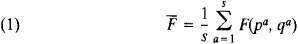or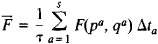where qa and pa are the values of the coordinates and momenta at times ta. In the limit s →∞, the sum becomes the integral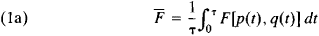The concept of the distribution function arises in a natural manner when the space of 6N dimensions is considered on whose axes the values of the coordinates and momenta of the particles in the system are plotted. Such a space is called a phase space. To each value of time t there correspond definite values of the q and p —that is, some point in the phase space. This point represents the state of the system at the given instant t. Suppose the phase space is divided into elements whose dimensions are small in comparison with the values of q and p characterizing the given state of the system but are still large enough that each element contains many points representing the state of the system at different times t. The number of such points in a volume element will then be approximately proportional to the magnitude of this element dpdq. If the proportionality factor is symbolized by sw(p, q), this number, for an element with center at some point (p, q), can be written in the form

(2) da = sw(p, q) dpdq

where

dpdq = dp1dq1dp2dq2 . . . dp3Ndq3N

is the volume of the selected element of the phase space. Because of the smallness of these volume elements, the average value (1) can be written

= (1/s) ʃ F da

that is

(3) (t) = ʃF[p(t), q(t)]w(p, q, t) dpdq

Here, integration is carried out over the coordinates throughout the entire volume of the system and over the momenta from−∞ to ∞. The function w(p, q, t) is the particle coordinate and momentum distribution function. Since the total number of points selected is s, the function w satisfies the normalization condition

(4) ʃw(p, q, t) dpdq = 1

It can be seen from (3) and (4) that w dpdq may be regarded as the probability that the system is located in the element dpdq of the phase space.

The distribution function introduced in this manner can be given another interpretation. For this purpose, we must consider simultaneously a large number of identical systems and assume that each point in the phase space reflects the state of one such system. The time average in (1) and (la) can then be understood as the average over the aggregate of these systems. This aggregate is known as an ensemble.

The above discussion is of a purely formal nature, since, according to (2), the finding of the distribution function requires knowledge of all p and q at all times—that is, requires the solution of the equations of motion with appropriate initial conditions. The basic principle of statistical mechanics, however, asserts that this function can be determined from general considerations for a system in a state of thermodynamic equilibrium. First of all, it can be shown, on the basis of the conservation of the number of systems during motion, that the distribution function is the integral of the system’s motion—that is, the function remains constant when p and q vary in accordance with the equations of motion (seeLIOUVILLES THEOREM).

When a closed system moves, its energy does not change. Consequently, the points in the phase space that represent the state of the system at different times must lie on some hypersurface corresponding to the initial value of the energy E. The equation of this surface has the form

H(p, q) = E

where H(p, q) is the energy of the system expressed in terms of the coordinates and momenta—that is, H(p, q) is the Hamilto-nian of the system. Since the motion of a many-particle system is extremely complicated, with time the points describing the state will be uniformly distributed over a surface of constant energy, just as, in the example given above, the grains in the vessel were uniformly distributed when mixed. Such a uniform distribution over a constant-energy surface is described by a distribution function of the form

(5) w(p, q) = Aδ[H(p, q)−E]

Here, δ[H(p, q)−E] is the delta function, which is nonzero only when H = E, that is, only on the surface, and A is a constant determined from normalization condition (4). Distribution function (5) is called the microcanonical function; it permits the average values of all physical quantities to be computed on the basis of equation (3) without solving the equations of motion.

In deriving expression (5) it was assumed that the energy of the system is the only quantity on which w depends that is conserved during the system’s motion. Of course, momentum and angular momentum are also conserved, but these quantities can be eliminated by assuming that the system in question is contained in a stationary box to which the particles can transfer momentum and angular momentum.

In practice, one usually considers not closed systems but macroscopic systems that are macroscopically small parts, or subsystems, of some closed system. The distribution function for a subsystem differs from (5) but does not depend on the specific character of the heat reservoir consisting of the remainder of the system. The distribution function of the subsystem can therefore be determined by assuming, for example, that the heat reservoir consists simply of N particles of an ideal gas. For clarity, the coordinates and momenta of these particles will be designated by Q and P, in contrast to the symbols q andp for the subsystem. The microcanonical distribution is then

w = Aδ[Σ(P2/2M) + H(p, q) = E]

Here, H(p, q) is the Hamiltonian of the subsystem, M is the mass of a gas particle, and summation is carried out over the components of the momenta of all particles in the heat reservoir. In order to find the distribution function of the subsystem, this expression must be integrated over the coordinates and momenta of the heat reservoir particles. It should be noted that the number of particles is much greater in the heat reservoir than in the subsystem. If N → ∞ and EIN is assumed to be constant and equal to 3kT/2, then the following expression is obtained for the distribution function of the subsystem:

(6) w(p,q) = exp{[F-H(p,q)]/kt}

The quantity T in this equation has the meaning of temperature, and k = 1.38 × 10–16 erg/degree is the Boltzmann constant. The condition EIN3kT/2 for the gas in the heat reservoir corresponds, as should be expected, to equation (13) for an ideal gas (see below). The normalization factor exp (FlkT) is determined from normalization condition (4):

(6a) exp (– F/kT) = Z = ʃ exp [ – H(p, q)/kT] dpdq

Distribution (6) is called the Gibbs canonical distribution, or simply the canonical distribution, and Z is called the partition function. In contrast to the microcanonical distribution, the energy of the system is not specified in the canonical distribution. The states of the system are concentrated in a thin layer of finite thickness around the energy surface corresponding to the average energy value. This circumstance means that energy can be exchanged with the heat reservoir. In other respects, the two distributions yield essentially the same results when applied to a specific macroscopic system. The only difference is that the average values are expressed in terms of the system’s energy when the microcanonical distribution is used and in terms of temperature when the canonical distribution is used.

Suppose a system consists of two noninteracting parts 1 and 2 with the Hamiltonians Hl and H2. For the entire system, then, H = Hi + H2, and, according to (6), the distribution function of the system can be decomposed into the product of the distribution functions for each part, so that these parts are statistically independent. This requirement, together with Liouville’s theorem, can be used to derive the canonical distribution without resorting to the microcanonical distribution.

Equation (6) is valid for systems that are described by classical mechanics. In quantum mechanics, the energy spectrum of a system of finite volume is discrete. The probability of a subsystem being in a state with energy En is given by an equation similar to

(6):

(7) wn = exp [(F – En)/kT]

In this case, the normalization condition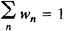can be written in the form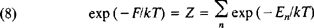The sum in expression (8) is taken over all states of the system, and the quantity Z is called the sum over states, or partition function, of the system.

For a system described with sufficient accuracy by classical mechanics, summation over states in equation (8) can be replaced by integration over the system’s coordinates and momenta. Moreover, to each quantum state there corresponds in the phase space a cell of volume (2πħ)3N where ħ is Planck’s constant. In other words, summation over n reduces to integration over dpdq/(2πħ)3N. It also should be taken into account that because of the identity of particles in quantum mechanics, the state of the system does not change when the particles are interchanged. Therefore, if we integrate over all p and q, the integral must be divided by the number of permutations of N particles, that is, by N!. Finally, the classical limit for the partition function has the form”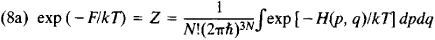This limit differs by a factor from the purely classical normalization condition (6a); as a result, there is an additional term in F.

The equations given above pertain to the case where the number of particles in the subsystem is specified. Suppose we choose as the subsystem a certain volume element of the system such that particles can escape from and return to the subsystem through the surface of the element. The probability of finding the subsystem in a state with energy En and number of particles Nn is then given by the equation of the Gibbs grand canonical distribution:

(9) wn = exp [(Ω – En – μNn)/kT]

Here, the additional parameter μ is the chemical potential, which determines the average number of particles in the subsystem, and the quantity Ω is determined from the normalization condition [see equation (11)].

Statistical interpretation of thermodynamics. The most important result of statistical mechanics is the establishment of the statistical meaning of thermodynamic quantities. This achievement permits the laws of thermodynamics to be deduced from the fundamental concepts of statistical mechanics and allows thermodynamic quantities to be calculated for specific systems. First of all, the thermodynamic internal energy is identified with the average energy of the system. The first law of thermodynamics then takes on an obvious interpretation as an expression of the law of conservation of energy during the motion of the particles making up the system.

Next, let the Hamiltonian of the system depend on some parameter λ, such as a coordinate of the wall of the vessel in which the system is contained, or an external field. The derivative ∂H/∂λ is then the generalized force corresponding to this parameter, and, after averaging, the quantity (∂H/∂λ)dλ gives the mechanical work performed on the system upon a change in this parameter. We now differentiate the expression E ̄ = ʃHw dpdq for the average energy of the system. Here, we take into account equation (6) and the normalization condition; we regard λ and T as variables and note that the quantity F is also a function of these variables. The following identity is then obtained: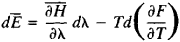As has been stated, the term containing is equal to the average work dA performed on the body. The second term is then the heat acquired by the body. Comparing this expression with the equation

dE = dA + TdS

which is a combined form of the first and second laws of thermodynamics for reversible processes (seeTHERMODYNAMICS, SECOND LAW OF), we find that T in (6) is in fact equal to the absolute temperature of the body, and the derivative ∂F/∂T is equal to the negative of the entropy 5. Consequently, F is the Helmholtz free energy of the system. This result reveals the statistical meaning of the free energy.

The statistical interpretation of entropy that follows from equation (8) is of particular importance. Formally, the summation in (8) is carried out over all states with energy En. In practice, however, because of the smallness of the energy fluctuations in the canonical distribution, only the relatively small number of states with energy close to the average are important. It is therefore reasonable to determine the number of these important states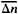by restricting the summation in (8) to the interval, replacing En by the average energy Ē, and removing the exponent from under the summation sign. The sum then gives, and (8) assumes the form:On the other hand, according to thermodynamics, F = Ē – TS. We thus obtain the relation between the entropy and the number of microscopic statesin the given macroscopic state—in other words, the relation between the entropy and the statistical weight of the macroscopic state, that is, the probability of the macroscopic state: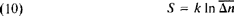At the absolute zero of temperature, any system is located in a definite ground state, so that= 1, and S = 0. This assertion expresses the third law of thermodynamics. It is important here that the quantum-mechanical equation (8) must be used in order to determine the entropy unambiguously. In purely classical statistics, the entropy is determined only to within an arbitrary term.

The interpretation of entropy as a measure of the probability of a state also holds with respect to arbitrary states—that is, states that are not necessarily equilibrium states. In a state of equilibrium, entropy has the maximum possible value under the given external conditions. Consequently, the equilibrium state is the state with the maximum statistical weight—that is, the most probable state. When a system passes from a nonequilibrium state to an equilibrium state, it passes from less probable states to more probable states; this circumstance clarifies the statistical meaning of the principle of entropy increase, according to which the entropy of a closed system can only increase.

Equation (8), which gives the relation between the free energy F and the partition function, is the basis for the calculation of thermodynamic quantities by the methods of statistical mechanics. In particular, the equation is used to construct the statistical theory of the electric and magnetic properties of matter. For example, to calculate the magnetic moment m of a system in a magnetic field, the partition function and free energy should be calculated. The magnetic moment is then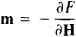where H is the strength of the external magnetic field.

In much the same way as with (8), the normalization condition for the grand canonical distribution [equation (9)] defines the thermodynamic potential Ω according to the equation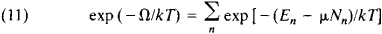The relation between this potential and the free energy is given by the equation

Ω = F – μN̄

The applications of statistical mechanics to the study of various properties of specific systems essentially reduce to the approximate calculation of the partition function, with allowance being made for the specific properties of the system.

In many cases, this task is simplified by applying the equiparti-tion law, which asserts that the specific heat cv (at constant volume v) of a system of interacting material particles—that is, particles executing harmonic vibrations—is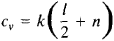where I is the total number of translational and rotational degrees of freedom and n is the number of vibrational degrees of freedom. The proof of the law is based on the fact that the Hamilto-nian H of such a system has the form H = K(pi) + U(qm), where the kinetic energy K is a homogeneous quadratic function of the l + n momenta pi, and the potential energy U is a quadratic function of the n vibrational coordinates qm. In the partition function Z defined in (8a), integration over the vibrational coordinates may extend from ∞ to + ∞ because of the rapid convergence of the integral. If we perform the change of variables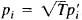and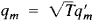we find that Z depends on temperature as T1/2+n, so that the free energy is F = – kT(l/2 + n)(ln T + const). Hence follows the expression given above for specific heat, since cv = – T∂2F/∂T2. Deviations from the equipartition law in real systems are due chiefly to quantum corrections, since the law is invalid in quantum statistical mechanics. There also exist corrections associated with the nonharmonic nature of the vibrations.

Ideal gas. The simplest object of investigation in statistical mechanics is the ideal, or perfect, gas, which is a gas so dilute that the interaction between its molecules may be ignored. The thermodynamic functions of such a gas can be completely calculated. The energy of the gas is simply equal to the sum of the energies of the individual molecules. This fact, however, does not mean that the gas molecules can be regarded as completely independent. Indeed, in quantum mechanics, even when forces of interaction between particles are absent, identical particles exert a certain influence on each other if they are in close quantum-mechanical states. This mutual influence is known as an exchange interaction. It may be ignored if there is an average of much less than one particle per state. This situation exists in a gas at a sufficiently high temperature; such a gas is called a nondegenerate gas. In actuality, ordinary gases consisting of atoms and molecules are non-degenerate at all temperatures at which they are still gaseous.

For a nondegenerate ideal gas, the distribution function can be written as the product of the distribution functions for the individual molecules. The energy of a molecule of a monatomic gas in an external field with the potential energy U(r) is p2/2M + U(r). By integrating (6) over the coordinates r(x, y, z) and momenta p(px, py, pz) of all the molecules but one, it is possible to find the number of molecules dN whose momenta lie in the intervals dpx, dpy and dpz and whose coordinates lie in the intervals dx,dy, ana dz: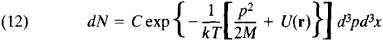where d3p = dpxdpydpz and d3x = dxdydz. This equation expresses the Maxwell-Boltzmann distribution (seeBOLTZMANN STATISTICS). If (12) is integrated over the momenta, a formula is obtained for the particle distribution with respect to coordinates in an external field. In the case of a gravitational field, the formula is known as the barometric formula. The distribution of velocities at each point in space is given by the Maxwellian distribution.

The partition function for an ideal gas can also be written as the product of identical terms corresponding to the individual molecules. For a monatomic gas, the summation in (8) reduces to integration over the coordinates and momenta—that is, the sum is replaced by the integral over d3p d3x/(2πħ)3 in accordance with the number of cells [with volume (2πħ)3] in the phase space of one particle. The free energy of N atoms of the gas is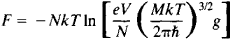where g is the statistical weight of the ground state of the atom (that is, the number of states corresponding to the atom’s lowest energy level), V is the volume of the gas, and e is the base of the natural logarithms. At high temperatures, g = (2J + 1)(2L = 1), where J is the spin and L is the orbital angular momentum of the atom (in units of ħ). It follows from the expression for free energy that the equation of state of an ideal gas, that is, the dependence of the pressure P on the particle density N/V and temperature has the form PV = NkT. The internal energy of a monatomic gas and its specific heat at constant volume turn out to be equal: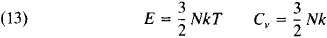The chemical potential of the gas is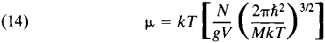It is characteristic that even for a nondegenerate gas—that is, a gas that obeys classical mechanics with sufficient accuracy—the expressions for free energy and chemical potential contain Planck’s constant ħ. This circumstance is ultimately due to the previously noted relation between entropy and the concept of the number of quantum states.

In the case of diatomic and polyatomic gases, the vibrations and rotation of molecules also make a contribution to the ther-modynamic functions. This contribution depends on whether the effects of the quantization of molecular vibrations and rotation are substantial. The distance between vibrational energy levels is of order ∆Ek = ħѡ, where ѡ is the characteristic frequency of the vibrations, and the distance between the first rotational energy levels is of order ∆Er = ħ2/2I, where I is the moment of inertia of the rotating particle (in the present case, a molecule).

Classical statistics is valid if the temperature is high enough so that

kT ≫ ∆E

In this case, in accordance with the equipartition law, rotation makes a constant contribution to the specific heat. This contribution is equal to k/2 for each rotational degree of freedom; for diatomic molecules, the contribution equals k. The vibrations make a contribution to the specific heat equal to k for each vibrational degree of freedom; thus, the vibrational specific heat of a diatomic molecule is k. The reason why the contribution of a vibrational degree of freedom is twice as great as that of a rotational degree of freedom is that the vibrating atoms in a molecule have not only kinetic but also potential energy.

In the opposite limiting case kT ≪ħѡ, the molecules are located in their ground vibrational state, whose energy is independent of temperature, so that the vibrations make no contribution at all to the specific heat. The same is true of the rotation of molecules under the condition kT ≪ ħ2/2l. As the temperature increases, molecules in excited vibrational and rotational states appear, and these degrees of freedom begin making a contribution to the specific heat. As the temperature continues to increase, they approach their classical limit.

Thus, the experimentally observed temperature dependence of the specific heat of gases can be explained by allowing for quantum effects. For most molecules, the values of the quantity ħ2/2kl, which characterizes the “rotation quantum,” are of the order of a few degrees or tens of degrees, for example, 85°K for H2, 2.4°K for O2, and 15°K for HC1. At the same time, the characteristic values of the quantity ħѡ/k for the “vibration quantum” are of the order of thousands of degrees, for example 6100°K for H2, 2700°K for O2, and 4100°K for HC1. The rotational degrees of freedom therefore begin contributing to the specific heat at much lower temperatures than do the vibrational degrees of freedom. Figure 1 shows the temperature dependence of the rotational (a) and vibrational (b) specific heats for a diatomic molecule (the rotational specific heat is for a molecule consisting of different atoms).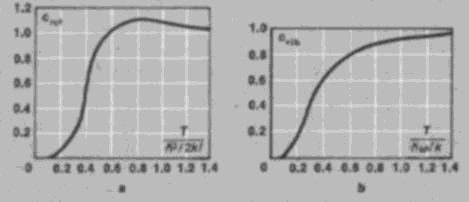Figure 1. Dependence of the rotational and vibrational parts of the specific heat of a diatomic gas on temperature T: (a) rotational part crot’ (b) vibrational part cvlb. The specific heats are in units of the classical values of specific heat.

Nonideal gas. An important achievement of statistical mechanics with regard to the thermodynamic quantities of a gas is the calculation of corrections that are associated with the interaction between the gas particles. From this standpoint, the equation of state of an ideal gas is the first term in the power series expansion for the pressure of a real gas in terms of particle density, since any gas behaves as an ideal gas at sufficiently low density. With increasing density, corrections to the equation of state that are associated with the interaction acquire importance. They lead to the appearance of terms with higher powers of particle density in the expression for pressure, so that the pressure is represented by a virial series of the form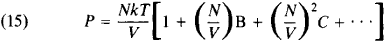The coefficients B, C, and so on depend on temperature and are called the second, third, and so on virial coefficients. Through the methods of statistical mechanics, these coefficients can be calculated if the law governing the interaction between the molecules of the gas is known.

The coefficients B, C, . . . describe the simultaneous interaction of two, three, or more molecules. For example, if the gas is monatomic and the interaction potential is U(r), then the second virial coefficient is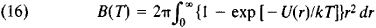B is of the order of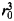where r0 is the characteristic atomic dimension, or more accurately, the range of the interatomic forces. This fact means that series (15) actually represents an expansion in powers of the dimensionless parameter Nr3/V, which is small for a sufficiently dilute gas. The interaction between the atoms of the gas is repulsive in character at short distances and attractive at long distances. Consequently, B > 0 at high temperatures, and B < 0 at low temperatures. The pressure of a real gas at high temperatures is therefore greater than the pressure of an ideal gas of the same density; at low temperatures the pressure of the real gas is less than that of the ideal gas. For example, for helium, B = – 3 × 10–23 cm3 at T = 15.3°K, and B = 1.8 × 10–23 cm3 at T = 510°K. For argon, B = –7.1 × 10–23 cm3 at T = 180°K, and B = 4.2 × 10–23 cm3 at T = 6000°K.

The values of the virial coefficients have been calculated for monatomic gases through the fifth coefficient. As a result, the behavior of the gases can be described over quite a broad range of densities (see alsoGASES).

Plasma. A plasma is a partially or completely ionized gas and therefore contains free electrons and ions. It is a special case of a nonideal gas. At a sufficiently low density, the properties of a plasma are close to those of an ideal gas. When the deviations of a plasma from an ideal gas are calculated, it must be taken into consideration that the electrons and ions interact electrostatically according to Coulomb’s law. The Coulomb forces decrease slowly with distance. Consequently, the simultaneous interaction of a large number of particles, rather than just two, must be taken into account in order to calculate the first correction for thermodynamic functions, since the integral in the second virial coefficient [equation (16)], which describes a binary interaction, diverges at large distances r between particles.

The Coulomb forces cause the distribution of the ions and electrons in a plasma to vary in such a way that the field of each particle is screened, that is, diminishes rapidly, at some distance called the Debye length. For the simplest case of a plasma consisting of electrons and singly charged ions, the Debye length rD is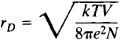where N is the number of electrons and e is the charge of an electron. All particles within the Debye length participate in the interaction simultaneously. As a result, the first correction to pressure is proportional not to (N/V)2, as in an ordinary gas, but to the lower power of density (N/V)312. The quantitative calculation is based on the remaining particles being distributed in the field of the selected electron or ion according to the Boltzmann distribution. When the first correction is taken into account, the equation of state consequently has the form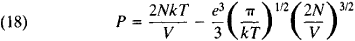It should be noted that since the number of electrons is equal to the number of ions, the total number of particles is 2N. Corrections of this kind also arise in the thermodynamic functions of electrolytes that contain free ions of the dissolved substances.

Liquids. In contrast to the situation for a gas, the interaction-related terms in the equation of state of a liquid are not small. The properties of a liquid are therefore strongly dependent on the specific character of the interaction between its molecules. The theory of liquids lacks a small parameter that could be used to simplify the theory. It is impossible to obtain any analytic formulas for the thermodynamic quantities of a liquid. One technique for overcoming this difficulty is to study a system consisting of a comparatively small number of particles—that is, a number of the order of a few thousand. In this case, an electronic computer can be used to carry out a direct solution of the equations of motion of the particles; the average values of all the quantities characterizing the system can thereby be determined without making additional assumptions. This technique permits investigation of the approach of the system to an equilibrium state. In another technique, the partition function for such a system consisting of a small number of particles is found by computer calculation of the integrals in the principal formula for the partition function; the Monte Carlo method is generally used here. Because of the small number of particles in the system, however, the results obtained with these two techniques have low accuracy when applied to real liquids.

The theory of liquids can also be constructed through the use of molecular distribution functions. If the distribution function w of a system is integrated over the momenta and coordinates of all particles but one, the single-particle space distribution function f1(r) is obtained. If w is integrated over the momenta and coordinates of all particles save two, the two-particle, or pair, distribution function f2(r1, r2) is obtained. Integration over all particles but three yields the three-particle distribution function F3(r1, r2, r3), and so on. The pair distribution function is a directly observable physical quantity—the elastic scattering of X rays and neutrons in a liquid, for example, can be expressed in terms of it.

By assuming that the distribution function of the entire system is given by the canonical distribution [equation (6)], an integral relation can be obtained that expresses the pair distribution function in terms of the three-particle function and the interaction potential of the particles. In the theory of the liquid state this exact relation is supplemented by some approximate relations that express the three-particle function in terms of the pair function (the single-particle function in a homogeneous liquid reduces to a constant). An equation for the pair function is consequently obtained; this equation is solved numerically. The supplementary relations are found on the basis of plausible physical considerations and are interpolative in character. As a result, theories making use of these relations may claim to give only a qualitative description of the properties of a liquid. Nonetheless, even such a qualitative description has considerable importance, since it evidences the generality of the laws of statistical mechanics (see alsoLIQUID).

Chemical equilibrium. Of great importance is the possibility afforded by statistical mechanics of calculating the constants of chemical equilibrium that determine the equilibrium concentrations of reactants. Thermodynamic theory yields the equilibrium condition that some linear combination of the chemical potentials of the substances must be equal to zero. In the case of a reaction between gases, the chemical potentials are determined by formulas similar to equation (14) for a monatomic gas, and the equilibrium constant can be calculated if the heat of reaction is known. Since the expressions for chemical potentials include Planck’s constant, quantum effects are important even for reactions between classical gases. The Saha equation, which gives the degree of ionization of a gas at equilibrium, is an important special case of the formulas of chemical equilibrium. (SeeEQUILIBRIUM, CHEMICAL.)

Degenerate gases. If the temperature of a gas is lowered at constant density, quantum-mechanical effects associated with the symmetry properties of the wave functions of a system of identical particles begin to show up. The gas “degenerates” (seeDEGENERATE GAS). For particles having half-integral spin, the wave function should change sign when any pair of particles is interchanged. This circumstance means, in particular, that no more than one particle can be in a single quantum state (the Pauli exclusion principle). Any number of particles with integral spin may be in the same state, but the invariability required in this case for the wave function upon interchange of particles leads here as well to a change in the statistical properties of the gas. Particles with half-integral spin are described by Fermi-Dirac statistics, and they are called fermions. Examples of fermions include electrons, protons, neutrons, deuterium atoms, and atoms of the light helium isotope 3He. Particles with integral spin are described by Bose-Einstein statistics and are known as bosons. These include hydrogen atoms, 4He atoms, and light quanta, that is, photons.

Suppose the average number of gas particles per unit volume with momenta lying in the interval d3p is npgd3p/(2πħ)3, so that np is the number of particles in one cell of the phase space. Here, g = 2j + 1, where J is the spin of the particle. It then follows from the canonical distribution that for ideal gases of fermions (plus sign) and bosons (minus sign)

(19) np = V{exp[(∈ = μ.)/kT]±1}

In this equation, ∊ = p2/2M is the energy of a particle with momentum p, and μ is the chemical potential, which is determined from the condition that the number of particles N in the system is constant: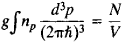Equation (19) becomes the formula for the Boltzmann distribution [equation (12)] when kT ≫ (ħ2/M)(N/V)2/3; the left side of this inequality becomes of the order of the right side at temperatures such that the de Broglie wavelength of particles moving with thermal velocity becomes of the order of the average distance between the particles. Thus, the lower the particle density in the gas (and the greater the mass of a particle M), the lower the temperatures at which degeneracy is exhibited.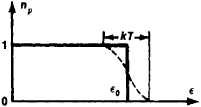Figure 2. Fermi-Dirac distribution function

In the case of fermions, as should be expected, np ≤ 1. This relation means that particles of a gas of fermions, or Fermi gas, have nonzero momenta even at T = 0, since only one particle can exist in a state with zero momentum. A more precise statement can be made in terms of the Fermi surface, which is a sphere in momentum space with radius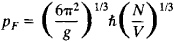For a Fermi gas at T = 0, np = 1 within the Fermi surface, and np = 0 outside this Fermi spnere. At finite but low temperatures, np varies gradually from 1 inside the sphere to 0 outside it; the width of the transition region is of order MkT/pF. Figure 2 shows the quantity np for a Fermi gas as a function of the energy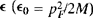. When the temperature of the gas changes, the state of the particles changes only in the transition layer. At low temperatures, the specific heat of a Fermi gas is proportional to T and is given by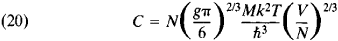In a Bose gas at T = 0, all particles are in the zero-momentum state. At sufficiently low temperatures, a finite fraction of the particles is in the state with p = 0; these particles form what is called the Bose-Einstein condensate. The other particles are in states with p ± 0, and their number is given by equation (19) with μ = 0. At a temperature of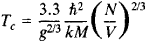a phase transition occurs in the Bose gas (see below). The fraction of the particles with zero momentum vanishes, and Bose-Einstein condensation disappears. The curve for specific heat as a function of temperature has a discontinuity at the point Tc. The momentum distribution of the particles at T > Tc is given by equation (19) with u. < 0. Figure 3 shows the Maxwellian, Fermi-Dirac, and Bose-Einstein (for T> Tc) distribution functions.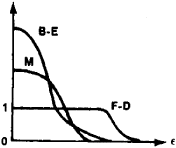Figure 3. Comparison of Maxwellian (M), Fermi-Dirac (F-D), and Bose-Einstein (B-D) distribution (unctions; the number of particles per single state with energy e is plotted along the axis of ordinates

Equilibrium electromagnetic radiation, which can be regarded as a gas consisting of photons, is a special case of the application of Bose-Einstein statistics. The relation between the energy of a photon and its momentum is given by the equation ∊ = ħω = pc, where c is the speed of light in a vacuum. The number of photons is not a specified quantity but is determined from the condition of thermodynamic equilibrium. The momentum distribution of photons is therefore given by equation (19) with μ, = 0 and ∊ = pc. The energy distribution in the frequency spectrum is obtained by multiplying the number of photons by the energy ∊, so that the energy density in the freqency interval is equal to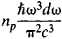where np is taken at ∊ = ħω. Planck’s equation for the spectrum of equilibrium (blackbody) radiation can be obtained in this manner (seePLANCK’S RADIATION LAW).

Crystal lattice. The application of statistical mechanics to the calculation of the thermodynamic functions of a crystal lattice is based on the circumstance that the atoms in the lattice undergo small vibrations about their equilibrium positions. The lattice can therefore be regarded as a set of coupled harmonic oscillators. The waves that can propagate in such a system obey a characteristic dispersion relation—that is, a characteristic dependence of the frequency o> on the wave vector k. In quantum mechanics, these waves may be regarded as a set of the elementary excitations, or quasiparticles, known as phonons.

A phonon has energy ħK and quasimomentum ftk. The chief difference between quasimomentum and momentum is that the energy of a phonon is a periodic function of quasimomentum with a period of order ħa, where a is the lattice constant. The quasimomentum distribution function of phonons is given by equation (19) for the Bose-Einstein distribution with μ = 0. Here, ∊ = ħω. Thus, if the function ω(k) is known, the specific heat of the lattice can be calculated. This function can be determined from experiments on the inelastic scattering of neutrons in the crystal (seeNEUTRON DIFFRACTION ANALYSIS) or can be calculated theoretically by specifying the values of the force constants that determine the interaction of the atoms in the lattice. At low temperatures, only low-frequency phonons are important. Such phonons correspond to quanta of ordinary sound waves, for which the relation between co and k is linear. Consequently, the specific heat of the crystal lattice is proportional to T3. At high temperatures, however, the law of equipartition of energy may be applied, so that the specific heat is independent of temperature and is equal to 3Nk, where N is the number of atoms in the crystal.

Metals. In metals, conduction electrons also contribute to the thermodynamic functions. The state of an electron in a metal is characterized by quasimomentum and, since electrons obey Fermi-Dirac statistics, their quasimomentum distribution is given by equation (19). At sufficiently low temperatures, the specific heat of the electron gas and, consequently, of the metal as a whole is therefore proportional to T. In contrast to the situation in a Fermi gas of free particles, the Fermi surface, near which the “active” electrons are concentrated, is not a sphere but a complex surface in quasimomentum space. The shape of the Fermi surface, like the dependence of energy on quasimomentum near this surface, can be determined experimentally, chiefly by investigating the magnetic properties of the metal. The shape of the Fermi surface can also be calculated theoretically by using the quasipotential model.

In superconductors (seeSUPERCONDUCTIVITY), the excited states of an electron are separated from the Fermi surface by a gap of finite width. As a result, the electronic specific heat is an exponential function of temperature. In ferromagnetic and antiferro-magnetic materials, spin waves, which are oscillations of the magnetic moments, also contribute to the thermodynamic functions.

In dielectrics and semiconductors, free electrons are absent at T = 0. At finite temperatures, charged quasiparticles appear in the substances. These quasiparticles are electrons with negative charge and an equal number of holes with positive charge. The combination of an electron and a hole in a bound state is a quasi-particle called an exciton. Another type of exciton is an excited state of an atom of a dielectric that moves through the crystal lattice.

Methods of quantum field theory. The methods of quantum field theory are of great importance for the solution of problems in quantum statistical mechanics, especially for the investigation of the properties of quantum fluids, electrons in metals, and magnetic substances. These methods were introduced into statistical mechanics comparatively recently.

A basic role in these methods is played by the Green’s function G of a macroscopic system, which is similar to the Green’s function of quantum field theory. C depends on the energy e and momentum p. The law of quasiparticle dispersion ∊(p) can be determined from the equation

(21) [G(∊, p)]–1 = 0

that is, the energy of a quasiparticle is determined by a pole of the Green’s function. A standard method exists for calculating Green’s functions in the form of a series of powers of the energy of interparticle interaction. Each term of the series contains multiple integrals over the energies and momenta of the Green’s functions of the noninteracting particles and can be given a graphical representation in the form of diagrams similar to the Feynman diagrams of quantum electrodynamics. Each diagram has a definite physical meaning. This fact makes it possible to identify in the infinite series the terms responsible for the phenomenon under study and to sum them. There also exists a diagrammatic technique of calculating Green’s temperature functions, which permit thermodynamic quantities to be calculated directly, without the introduction of quasiparticles.

Close to the methods of quantum field theory in many regards are extensions of the methods mentioned above in the section on liquids. Here, the multiparticle distribution functions are applied to quasiparticles. The use of these functions always is based on approximate “uncoupling”—that is, the expression of a function of higher order in terms of lower-order functions.

Phase transitions. When external parameters, such as pressure or temperature, vary continuously, the properties of the system may vary discontinuously at certain values of the parameters —that is, a phase transition may occur. Phase transitions are divided into transitions of the first and second orders. First-order transitions are accompanied by a latent heat of transition and by an abrupt change in volume; melting is an example of such a transition. In second-order transitions, there is no latent heat or abrupt change in volume; an example is the transition to the superconducting state. The statistical theory of phase transitions forms an important but still far from fully developed branch of statistical mechanics. The greatest difficulty for theoretical investigation is posed by the properties of a substance near the line of a second-order transition and near the critical point of a first-order transition. From a mathematical standpoint, the thermodynamic functions of the system have singularities here. Distinctive critical phenomena occur near these points. At the same time, fluctuations anomalously arise here, and the approximate methods of statistical mechanics considered above are inapplicable. An important role is therefore played by a small number of exactly solvable models in which there are transitions; an example is the Ising model.

Fluctuations. Statistical mechanics is based on the fact that the physical quantities characterizing macroscopic systems are, to a high degree of accuracy, equal to their average values. This equality is nonetheless approximate. In actuality, all quantities undergo fluctuations, or small random deviations from their average values. The existence of fluctuations is of fundamental importance, since it demonstrates the statistical character of thermodynamic regularities. Moreover, fluctuations play the role of noise, which interferes with physical measurements and limits their accuracy.

The fluctuations of some quantity x about its average value are characterized by the mean square fluctuation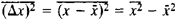In most cases, the quantity x undergoes fluctuations of order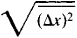Fluctuations of a much greater magnitude occur extremely infrequently. If the distribution function of the system is known, the mean square fluctuation can be calculated in precisely the same way as the average value of any physical quantity. Small fluctuations of thermodynamic quantities can be calculated by using the statistical interpretation of entropy. According to (10), the probability of a nonequilibrium state of a system with entropy S is proportional to exp (S/k). We consequently have the formula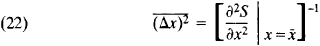For example, the mean square fluctuations of the volume and temperature of a substance are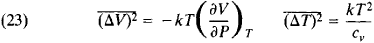It is evident from these formulas that the relative fluctuations of volume and the fluctuations of temperature are inversely proportional to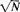where N is the number of particles in the system. This result ensures that the fluctuations are small for macroscopic bodies.

The relation between the fluctuations of different quantities xi and xk is characterized by the function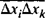If the fluctuations of the quantities xi and xk are statistically independent, then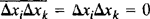.

We can also take xi and xk to be the values of some single quantity, such as density, at different points in space. The function can then be understood as a space correlation function. As the distance between the points increases, the correlation function tends toward zero (usually exponentially), since fluctuations at distant points in space occur independently. The distance at which the function substantially decreases is called the correlation distance.

The time dependence of fluctuations and the spectral distribution of fluctuation noise are described by the time correlation function φ(t), in which the fluctuations of a quantity at different times t are averaged: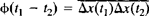An important role is played in fluctuation theory by the fluctuation-dissipation theorem, which relates the fluctuations in a system to the change in its properties under the action of certain external influences. The simplest relation of this type can be obtained by considering the fluctuations of a harmonic oscillator with potential energy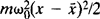where m is the mass of the oscillator and ω0 is its natural frequency. A calculation using equation (22) gives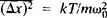On the other hand, if a force f acts on the oscillator, the average value x is changed by the amount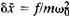SO that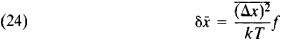and the fluctuation of x is related to the perturbation due to f. In the general case, the fluctuation-dissipation theorem is applicable if for x there exists a generalized force f that appears in the system’s energy operator, or Hamiltonian operator (seeQUANTUM MECHANICS) in the form of the term – fx̂, where is a quantummechanical operator corresponding to the quantity x. The incorporation of the force f leads to a change of δx̄ in the average value If f is time-dependent as exp (– iωt), this change can be written in the form

δx̄ = α(ω)f

The complex quantity α((ω) is called the generalized susceptibility of the system. The theorem asserts that the Fourier transform of the correlation function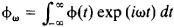can be expressed in terms of α in the following manner: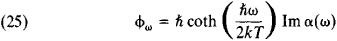where Im represents the imaginary part of the function. The Ny-quist theorem is a special case of (25).

Nonequilibrium processes. Physical kinetics, which is the branch of statistical mechanics that studies processes in systems in non-equilibrium states, is acquiring increasing importance. Here, two formulations of the problem are possible. First, a system may be considered in some nonequilibrium state, and the system’s transition to an equilibrium state may be observed. Second, a system may be considered whose nonequilibrium state is maintained by external conditions. An example is a system in which a temperature gradient is predetermined or in which an electric current is flowing. Another example is a system located in a variable external field.

If the departure from equilibrium is small, the nonequilibrium properties of the system are described by “kinetic,” or transport, coefficients. Examples of such coefficients are viscosity, thermal conductivity, diffusivity, and the electrical conductivity of metals. The quantities characterized by these coefficients satisfy the symmetry principle for the coefficients, which expresses the symmetry of the equations of mechanics under a change in the sign of time (seeONSAOER THEOREM). By virtue of this principle, for example, the electrical conductivity of a crystal is described by a symmetric tensor.

The description of markedly nonequilibrium states and the calculation of the mentioned coefficients are carried out by means of a transport equation, which is an integro-differential equation for the single-particle distribution function (in the quantum case, for a single-particle density matrix). Such a closed equation, that is, an equation that does not contain other quantities, cannot be obtained in a general form. The derivation of the equation must make use of the small parameters available for the given specific problem.

The most important example of a transport equation is the Boltzmann equation, which describes the establishment of equilibrium in a gas through intermolecular collisions. The Boltzmann equation is valid for sufficiently rarefied gases, where the mean free path is large compared with the distances between the molecules. The specific form of the equation depends on the effective cross section for the scattering of the molecules by each other. If this cross section is known, the equation can be solved by expanding the unknown function in a series of orthogonal polynomials (seeORTHOGONAL SYSTEM OF FUNCTIONS). The kinetic coefficients of a gas can thus be calculated on the basis of the known laws governing the intermolecular interaction. The Boltzmann equation takes into account only binary collisions between molecules and describes only the first nonvanishing term of the expansion of the coefficients in terms of gas density. A more exact equation that also allows for triple collisions has been found. This achievement has made it possible to calculate the next term of the expansion.

The derivation of the transport equation for a plasma poses a particular problem. Because of the slow decrease in Coulomb forces with distance, the screening of these forces by the remaining particles is considerable, even when binary collisions are considered.

The nonequilibrium states of solids and quantum fluids at low temperatures may be regarded as the nonequilibrium states of a gas of the corresponding quasiparticles. Transport processes in such systems are therefore described by the transport equations for quasiparticles, which take into account the collisions between the quasiparticles and the processes of the quasiparticles’ mutual transformation.

New possibilities have been opened up by the introduction of the methods of quantum field theory into physical kinetics. The kinetic coefficients of a system may be expressed in terms of its Green’s function, for which there exists a general method of calculation using diagrams. In many cases, this approach makes it possible to obtain the coefficients without explicit use of the transport equation and to investigate the nonequilibrium properties of the system, even when the conditions of applicability of the transport equation are not satisfied.

Main landmarks in the development of statistical mechanics. Statistical mechanics is based wholly on concepts of the atomic structure of matter. The initial period of development of statistical mechanics therefore coincides with the development of atomistic concepts (seeATOMISM). The development of statistical mechanics as a branch of theoretical physics began in the mid-19th century. In 1859, J. Maxwell determined the velocity distribution function of gas molecules. Between 1860 and 1870, R. Clausius introduced the concept of mean free path and related it to the viscosity and thermal conductivity of a gas. At approximately the same time, L. Boltzmann generalized the Maxwell distribution to the case where a gas is in an external field, proved the theorem of equipar-tition of energy, derived the transport equation, gave a statistical interpretation of entropy, and showed that the law governing the increase of entropy follows from the transport equation. The construction of classical statistical mechanics was completed by 1902 in the works of J. W. Gibbs.

The theory of fluctuations was developed in 1905 and 1906 by M. Smoluchowski and A. Einstein. M. Planck’s derivation in 1900 of the law of energy distribution in a blackbody emission spectrum initiated the development of both quantum mechanics and quantum statistical mechanics. In 1924, S. Bose found the momentum distribution of light quanta and related it to the Planck distribution. A. Einstein generalized the Bose distribution to gases with a specified number of particles. In 1925, E. Fermi obtained the distribution function for particles obeying the Pauli exclusion principle, and P. A. M. Dirac established the relation of this distribution and the Bose-Einstein distribution to the mathematical apparatus of quantum mechanics. The subsequent development of statistical mechanics in the 20th century involved the application of its basic principles to the investigation of specific problems.

### REFERENCES

Classical works
Boltzmann, L. Lektsii po leorii gazov. Moscow, 1956. (Translated from German.)
Boltzmann, L. Stat’i i rechi. Moscow, 1970. (Translated from German.)
Gibbs, J. W. Osnovnye printsipy statistkheskoi mekhaniki. Moscow-Leningrad, 1946. (Translated from English.)
Textbooks
Ansel’m, A. I. Osnovy statisticheskoi fiziki i lermodinamiki. Moscow, 1973.
Leontovich, M. A. Statisticheskaia fizika. Moscow-Leningrad, 1944.
Landau, L. D., and E. M. Lifshits. Teoreticheskaia fizika, vol. 5, 2nd ed. Moscow, 1964.
Mayer, J., and M. Goeppert-Mayer. Statisticheskaia mekhanika. Moscow, 1952. (Translated from English.)
Kittel, C. Kvantovaia teoriia tverdykh tel. Moscow, 1967. (Translated from English.)
Hill, T. Statisticheskaia mekhanika: Printsipy i izbrannye prilozheniia. Moscow, 1960. (Translated from English.)
Huang, K. Statisticheskaia mekhanika. Moscow, 1966. (Translated from English.)
Specialized Literature
Abrikosov, A. A., L. P. Gor’kov, and I. E. Dzialoshinskii. Metody kvantovoi teoriipolia v statistkheskoifizike. Moscow, 1962.
Bogoliubov, N. N. Problemy dinamicheskoi teorii v statistkheskoi fizike. Moscow-Leningrad, 1946.
Gurevich, L. E. Osnovy fizicheskoi kinetiki. Leningrad-Moscow, 1940.
Silin, V. P. Vvedenie v kineticheskuiu teoriiu gazov. Moscow, 1971.
Fizika prostykh zhidkostei: Sb. Moscow, 1971. (Translated from English.)

L. P. PITAEVSKII

## statistical mechanics

[stə′tis·tə·kəl mi′kan·iks]
(physics)
That branch of physics which endeavors to explain and predict the macroscopic properties and behavior of a system on the basis of the known characteristics and interactions of the microscopic constituents of the system, usually when the number of such constituents is very large. Also known as statistical thermodynamics.
McGraw-Hill Dictionary of Scientific & Technical Terms, 6E, Copyright © 2003 by The McGraw-Hill Companies, Inc.
References in periodicals archive ?
A lattice hydrodynamic model based on delayed feedback control considering the effect of flow rate difference, Physica A: Statistical Mechanics and its Applications 479: 478-484.
Deppman, "Non extensive thermodynamics for hadronic matter with finite chemical potentials," Physica A: Statistical Mechanics and its Applications, vol.
Cai, "Empirical analysis of a scale-free railway network in China," Physica A: Statistical Mechanics and its Applications, vol.
Bentes, "Long memory volatility of gold price returns: how strong is the evidence from distinct economic cycles?" Physica A: Statistical Mechanics and its Applications, vol.
Ding, "Invulnerability of scale-free network against critical node failures based on a renewed cascading failure model," Physica A: Statistical Mechanics and Its Applications, vol.
Fu, "Temporal-spatial diversities of long-range correlation for relative humidity over China," Physica A: Statistical Mechanics and its Applications, vol.
Tanaka, "Study of bottleneck effect at an emergency evacuation exit using cellular automata model, mean field approximation analysis, and game theory," Physica A: Statistical Mechanics and Its Applications, vol.
A network method to investigate the effects of network structure on stock returns, Physica A: Statistical Mechanics and its Applications, 436, 224-235.
Gibbs' second magnum opus, Elementary Principles in Statistical Mechanics, Developed With Especial Reference to the Rational Foundation of Thermodynamics, which appeared in 1902, changed all that.
Gao, "Non-traditional spectral clustering algorithms for the detection of community structure in complex networks: a comparative analysis," Journal of Statistical Mechanics: Theory and Experiment, vol.
Wang, "Effect of randomization in the Biham-Middleton-Levine traffic flow model," Journal of Statistical Mechanics: Theory and Experiment, vol.
Frank, "A Langevin approach for the microscopic dynamics of nonlinear Fokker-Planck equations," Physica A: Statistical Mechanics and its Applications, vol.

Site: Follow: Share:
Open / Close